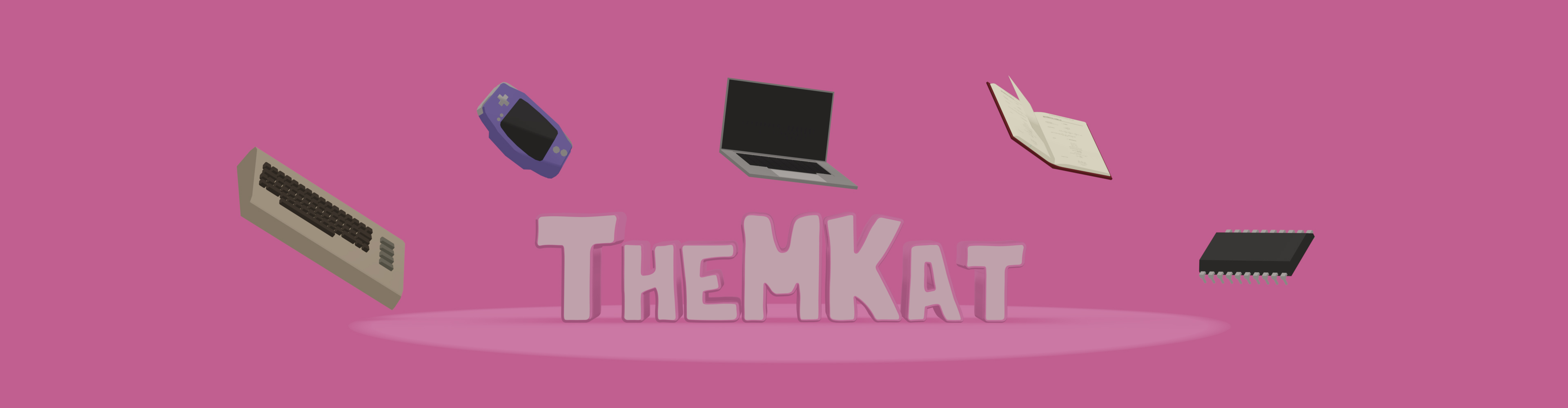## Bit tricks - Counting the number of 1 bits (Kernighan algorithm)

Published Sat Oct 22 2022

It's been too long since the last bit tricks article, so I thought I would share another one! This time we will look at a simple fun bit trick to count the number of 1 bits in a binary string (e.g, a 32 bit integer). This algorithm was discovered by Brian Kernighan of Bell Labs and C fame. Unlike the previous algorithm presented in my bit tricks series, this one requires some branching.

If you are interested in more bit tricks after reading this article, I also have an article on absolute value without branching.

I will refer to the structures we are working on as binary strings. Binary strings in this context is just a series of bits. It can be an integer, character, long, floating point number etc. For this algorithm you would off course have to convert it to a structure we could work with like an integer, but lucky for us, all of the previous types can be casted to something suitable.

## The algorithm

### Pseudo code and basics

How do we count the number of 1 bits in a binary string? One possible solution is to clear the lowest 1 bit in each step, and then count the number of steps needed for the input to become 0. This is the algorithm we will look at today. We will work on unsigned ints here, but it could easily have been chars, unsigned longs or something else. Unsigned because we want to count the number of bits, even for negative numbers, so we would have to cast signed variants to unsigned types. I find it easier to work on numbers where the lowest possible value is 0 in this case. The basic algorithm looks like this in a while-loop variant:

unsigned int x = input;
unsigned int c = 0;
while (x > 0) {
x = x & (x - 1);
c++;
}


Or as a for-loop variant:

unsigned int x = input;
unsigned int c;
for (c = 0; x > 0; c++) {
x = x & (x - 1);
}


(x = x & (x - 1) could also be written as x &=(x - 1))

So we do an operation x & (x - 1) on an unsigned number (can also be a char, long etc.) until it is 0. So by doing this operation on x a number of times, we have the number of 1 bits stored in c (c stands for count). In short x & (x - 1) removes the lowest one bit in the binary number in each operation. How does this work?

### Explaining the base step

We know from the previous section that x & (x - 1) removes the lowest 1 bit in each step. The first time you see it, it can be a bit confusing. I think it is best explained by looking at a few examples to gain some intuition. First of all, remember that the AND-operation (&) only returns 1 if both bits are one. AND has the following truth-table (a and b are the input bits, result is the result of the operation):

a b result
0 0 0
0 1 0
1 0 0
1 1 1

(In case you haven't looked at binary operations in some time: Doing an AND operation on a binary string with multiple bits is simply doing the operation on each bit pair. first bit of a AND first bit of b, second bit of a AND second bit of b, …, last bit of a AND last bit of b.)

To make the examples simple, they will be 8 bit data aka one byte. In my view, the easiest way to understand this algorithm is to do some binary subtractions yourself, and see how they behave. By looking at both x and x-1, we then see that the lowest one bit in the result has to be cleared.

#### Example input: 4

Let us first look at the number 4 (00000100). What is the result of doing the operation above:

x:           00000100
x - 1:       00000011
x & (x - 1): 00000000


By looking at x and x-1, we see that the and-operation clearly has to have the result 0. The loop above would only run for one iteration here, and result in c=1 (number of 1 bits equal to 1).

#### Example input: 7

x:           00000111
x - 1:       00000110
x & (x - 1): 00000110


#### Example input: 25

x:           00011001
x - 1:       00011000
x & (x - 1): 00011000


#### Example input: 24

x:           00011000
x - 1:       00010111
x & (x - 1): 00010000


If you try to do the step again you have x (00010000), x-1 (00001111) and then x&(x-1) = 0. For input number 24, we would then get c=2.

## Where can this be useful?

The most obvious place I can think of this as being useful is when you have encoded data into bits. One example include saving the on-off state of something like Conway's game of life into bits (each bit is an on-off cell in the grid). Then you can simply mask the data, and then count the number of bits to find adjacent neighbors. Choosing an algorithm for that case depends on if you are optimizing for speed of program size.

That being said, encoding into bits like this is not something most developers does today. The exception is off course some embedded programmers, and those of us who dabble in retro computing.